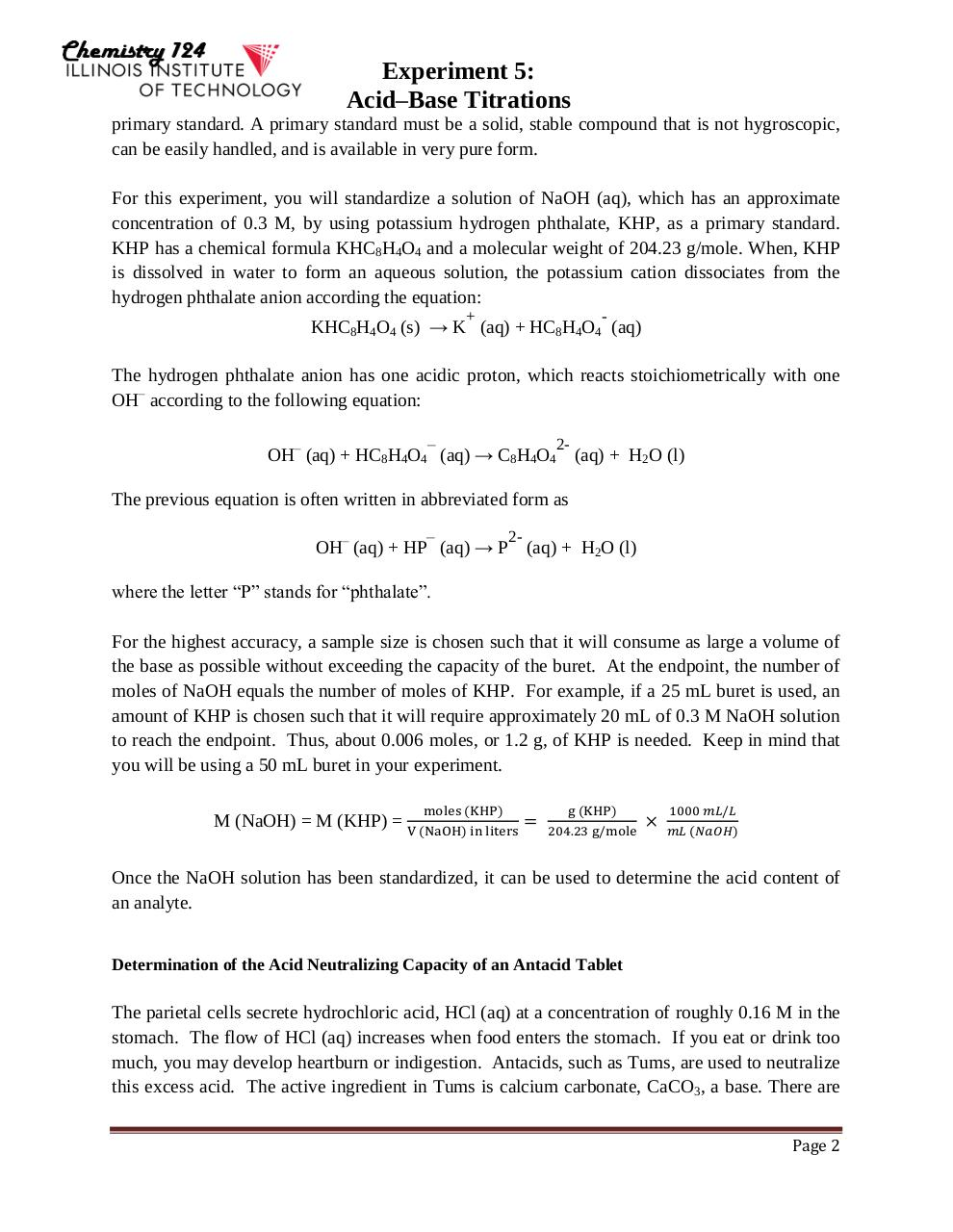# Lab 5 Titrations Fall 2015(1).pdfPage 1 2 3 4 5 6 7 8 9 10 11 12 13

#### Text preview

Experiment 5:
Acid–Base Titrations
primary standard. A primary standard must be a solid, stable compound that is not hygroscopic,
can be easily handled, and is available in very pure form.
For this experiment, you will standardize a solution of NaOH (aq), which has an approximate
concentration of 0.3 M, by using potassium hydrogen phthalate, KHP, as a primary standard.
KHP has a chemical formula KHC8H4O4 and a molecular weight of 204.23 g/mole. When, KHP
is dissolved in water to form an aqueous solution, the potassium cation dissociates from the
hydrogen phthalate anion according the equation:
+

-

KHC8H4O4 (s) → K (aq) + HC8H4O4 (aq)
The hydrogen phthalate anion has one acidic proton, which reacts stoichiometrically with one
OH– according to the following equation:

2-

OH– (aq) + HC8H4O4 (aq) → C8H4O4 (aq) + H2O (l)
The previous equation is often written in abbreviated form as

2-

OH– (aq) + HP (aq) → P (aq) + H2O (l)
where the letter “P” stands for “phthalate”.
For the highest accuracy, a sample size is chosen such that it will consume as large a volume of
the base as possible without exceeding the capacity of the buret. At the endpoint, the number of
moles of NaOH equals the number of moles of KHP. For example, if a 25 mL buret is used, an
amount of KHP is chosen such that it will require approximately 20 mL of 0.3 M NaOH solution
to reach the endpoint. Thus, about 0.006 moles, or 1.2 g, of KHP is needed. Keep in mind that
you will be using a 50 mL buret in your experiment.
M (NaOH) = M (KHP) =

moles (KHP)
V (NaOH) in liters

=

g (KHP)
204.23 g/mole

×

1000 𝑚𝐿/𝐿
𝑚𝐿 (𝑁𝑎𝑂𝐻)

Once the NaOH solution has been standardized, it can be used to determine the acid content of
an analyte.
Determination of the Acid Neutralizing Capacity of an Antacid Tablet

The parietal cells secrete hydrochloric acid, HCl (aq) at a concentration of roughly 0.16 M in the
stomach. The flow of HCl (aq) increases when food enters the stomach. If you eat or drink too
much, you may develop heartburn or indigestion. Antacids, such as Tums, are used to neutralize
this excess acid. The active ingredient in Tums is calcium carbonate, CaCO3, a base. There are
Page 2